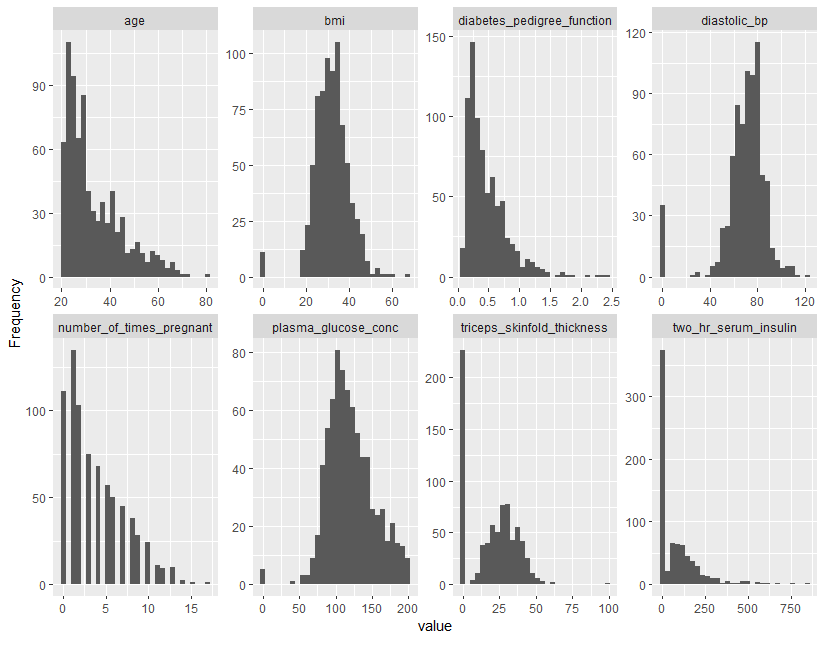Want to share your content on R-bloggers? click here if you have a blog, or here if you don't.Data exploration is an important part of the modeling process. It can also take up a fair amount of time. The awesome DataExplorer package in R aims to make this process easier. To get started with DataExplorer, you’ll need to install it like below:

```
install.packages("DataExplorer")

```

Let’s use DataExplorer to explore a dataset on diabetes.

```
library(DataExplorer)

# fix column names
names(diabetes_data) <- c("number_of_times_pregnant", "plasma_glucose_conc", "diastolic_bp", "triceps_skinfold_thickness", "two_hr_serum_insulin", "bmi", "diabetes_pedigree_function", "age", "label")

# create report
create_report(diabetes_data)

```

Running the create_report line of code above will generate an HTML report file containing a collection of useful information about the data. This includes:

• Basic statistics, such as number of rows and columns, number of columns with missing data, count of continuous variables vs. discrete, and the total memory allocation
• Data type for each field
• Missing data percentages for each column
• Univariate distribution for each column
• QQ plots
• Correlation analysis
• PCA

• That’s right – a single line of code can generate all of the above for a given dataset! It’s also possible to get each of these pieces individually. For example, in a single line of code, we can generate histograms for all the numeric variables in the dataset.

```
plot_histogram(diabetes_data)

```Similarly, we can get bar plots for all categorical variables in the dataset

```
plot_bar(diabetes_data)

```

Here’s an example getting the correlation plot:

```
plot_correlation(diabetes_data)

```### Configuring the report

It’s also possible to make adjustments to the output generated by create_report. For example, if you don’t want the QQ plots, you could set add_plot_qq = FALSE

```

create_report(config = config)

```

### One hot encoding

DataExplorer also comes with a function to perform one hot encoding. You can one hot encode all the categorical variables in the dataset by passing the data frame name to the dummify function. In this case, we don’t have any categorical variables to encode, so the function will generate a warning.

```
dummify(diabetes_data)

```

## Conclusion

That’s all for this post! If you liked this post, consider buying me a coffee. Check out my other R posts by clicking here.

The post Faster data exploration with DataExplorer appeared first on Open Source Automation.